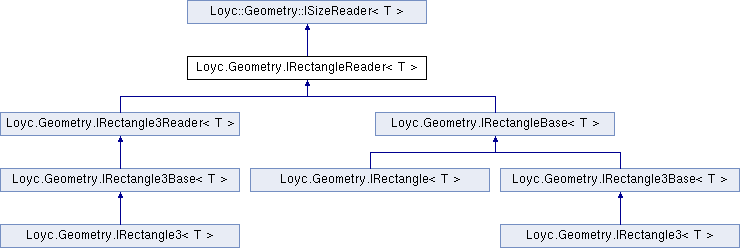Enhanced C# Language of your choice: library documentation
Loyc.Geometry.IRectangleReader< T > Interface Template Reference

Represents a read-only 2D rectangle. More...

Source file:
Inheritance diagram for Loyc.Geometry.IRectangleReader< T >:## Remarks

The relationship between X1, X2 and Width, and the relationship between Y1, Y2 and Height, depends on whether the object represents a normal (traditional Windows) rectangle, a bounding rectangle, or a pair of points. A traditional rectangle defines its Height as Y2 - Y1, and its Width as X2 - X1. A bounding rectangle is defined slightly differently: the Width is X2 - X1 + e, and the Height is Y2 - Y1 + e, where e is an infitessimal value of type T (e=1 if T is an integer type).

Also, a traditional rectangle is stored as a starting point (X1, Y1) and a size (Width, Height), while a bounding rectangle stores X2 and Y2 instead, not the Width and Height.

Finally, this interface could simply represent a pair of points. In that case, Width and Height return the absolute value of X2-X1 and Y2-Y1 respectively.

A rectangle may or may not require X2 >= X1 and Y2 >= Y1. If X1>X2 or Y1>Y2, the rectangle is said to be "not normalized" and the RectangleExt.IsNormal<Rect, T>(Rect) extension method returns false.

## Properties

X1` [get]`

Y1` [get]`

X2` [get]`

Y2` [get]`Properties inherited from Loyc.Geometry.ISizeReader< T >
Width` [get]`
Gets the width of a rectangle (the difference between X coordinates). More...

Height` [get]`
Gets the height of a rectangle (the difference between Y coordinates). More...